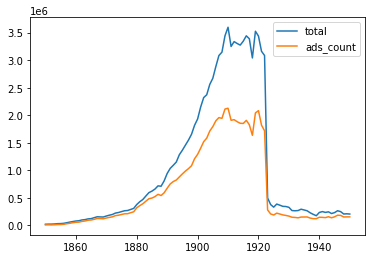## Aims

This notebook will go through the process of creating a sample for input to a machine learning model. The code is pretty minimal. A good chunk of the notebook is asking questions about the best apporach.

## Creating a sample for the period 1850-1950

We have a few questions to consider when sampling:

• What do we want the model to be able to do well at?
• Newspaper navigator training data
• Models on models on models (using outputs from other models)
• How much time can we put into annotating?

First we import some modules from nnanno and Path from the pathlib from the Python standard library which makes working with paths delightful.

from pathlib import Path

from nnanno.sample import *
from nnanno.annotate import *


We create an nnSampler instance which we can use to creat our sample

sampler = nnSampler()


### Choosing paramters for sampling

One of the first decisions we need to make is which paramters we'll use to create our sample. We can access the 'population' of the Newspaper Navigator data via population. This returns a Pandas DataFrame containing the number of ads for each year. We can quickly plot this to see the distribution over time

sampler.population[["total", "ads_count"]].plot()

<AxesSubplot:>We can see that the number of adverts grows over time and drops off sharply. This trend broadly follows the same pattern as the overall dataset.

We have two questions when creating a sample to train a model to classify images of ads as 'visual' or 'not visual':

• How much to sample?
• How to sample?

For the first question, we'll create a sample of ~1000 images. Hopefully, this will be a good balance between generating a big enough training dataset and not having to annotate too much. Since we're going to be annotating binary labels, the cognitive load of annotating becomes much lower, which should also help make a higher number of annotations relatively quick to do. Whether this number is enough to train a good classifier will depend on what we're trying to label. There may be a temptation to do all of the annotations initially, but we'll often learn things about our data from training a model, so we may want to try and get to this stage sooner. We can come back to the sampling/annotation step if we need.

How to sample? We currently have a few main options:

• Sample for every year or take a sample for every n years
• Sample a specific number for each year, i.e. 100 examples per year
• Sample a fraction from each year, i.e. 1% per year

Since we are working with an uneven distribution of samples, we could reasonably choose to sample a fraction for each year. However, because we are training a computer vision model with this data, we may want to help ensure our model works equally well for every year by showing an even number of examples for each year. Whether this (i.e. the period of the training data is vital for accuracy on all periods) is essential or not, we'll begin to look at in the following notebook.

The time it takes to generate this sample will depend on your connection speed. If you have previously requested the same data the results will be cached making the request quicker.

df = sampler.create_sample(1000, "ads", step=10, year_sample=False)


We now have our sample inside a dataframe. We create a folder to keep our data (semi) organised

Path("data").mkdir()


We can use create_label_studio_json to turn this sample into a json file that we can use for creating annotation tasks in the label-studio annotation software.

create_label_studio_json(sampler, "data/ad_tasks.json", size=(400, 400))


This command returns a json file containing the IIIF links (with specified) sizes that we can use to load images into label-studio.

We'll create a new label studio project using the label-studio init command. See the label studio documentation for more details on options for setup. In this example I used the GUI to load in the ads_task.json file for creating the tasks. "Tasks" here means the images we are going to annotate.

We use the following XML file as our 'label conig'. Again the docs for label studio give more information on how to create these configs.

<View>
<Image name="image" value="\$image"/>
<Choices name="choice" toName="image" showInLine="true">
<Choice value="visual" background="blue"/>
<Choice value="text_only" background="green" />
</Choices>
</View>


The next step is to annotate. For this task the annotations didn't take too long (an hour or so) since the labels are quite 'obvious' to a human eye and since we only have two options to choose from.

When we have completed the annotations we can export them as a CSV file from label studio. We can then use the load_annotations function to parse this CSV into a Pandas DataFrame

df = load_annotations_csv("data/results.csv")

Now we have loaded our annotations, it's likely we want to download them locally. We can do this using the sampler.download_sample method. This can be useful if you are working locally when doing the annotations but want to work in the cloud to train a model. The annotations csv is small enough to store in version control and the images themselves can be download once in the cloud.
sampler.download_sample("data/images", original=True, df=df)